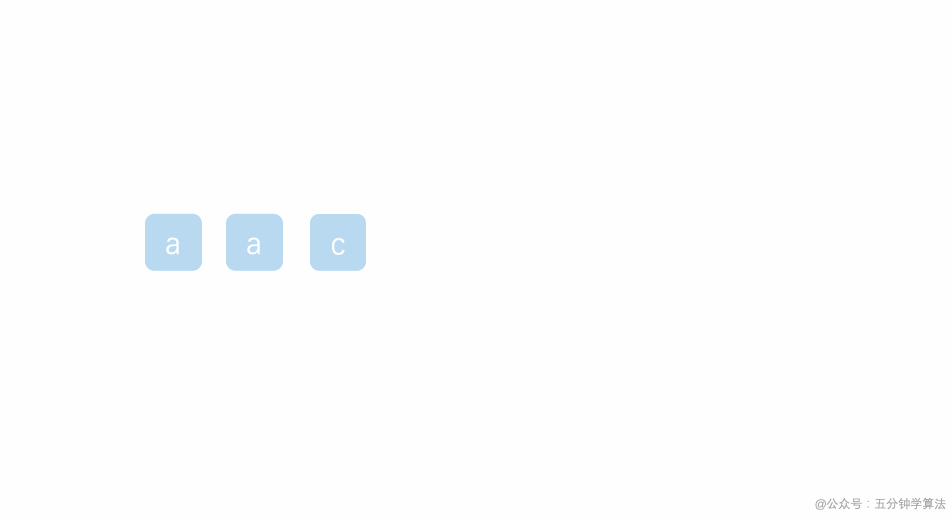## LeetCode 第 131 号问题：分割回文串 有更新！

Published on with 309 views

# LeetCode 第 131 号问题：分割回文串

### 题目描述

``````输入: "aab"

[
["aa","b"],
["a","a","b"]
]
``````

### 题目解析

1. 分割为 a + ac
2. 分割为 a + a + c，分割后，得到一组结果，再回溯到 a + ac
3. a + ac 中 ac 不是回文串，继续回溯，回溯到 aac
4. 分割为稍长的回文串，分割为 aa + c 分割完成得到一组结果，再回溯到 aac
5. aac 不是回文串，搜索结束

### 动画描述### 代码实现

``````class Solution {
List<List<String>> res = new ArrayList<>();

public List<List<String>> partition(String s) {
if(s==null||s.length()==0)
return res;
dfs(s,new ArrayList<String>(),0);
return res;
}

public void dfs(String s,List<String> remain,int left){
if(left==s.length()){  //判断终止条件
res.add(new ArrayList<String>(remain));  //添加到结果中
return;
}
for(int right=left;right<s.length();right++){  //从left开始，依次判断left->right是不是回文串
if(isPalindroom(s,left,right)){  //判断是否是回文串
remain.add(s.substring(left,right+1));   //添加到当前回文串到list中
dfs(s,remain,right+1);  //从right+1开始继续递归，寻找回文串
remain.remove(remain.size()-1);  //回溯，从而寻找更长的回文串
}
}
}
/**
* 判断是否是回文串
*/
public boolean isPalindroom(String s,int left,int right){
while(left<right&&s.charAt(left)==s.charAt(right)){
left++;
right--;
}
return left>=right;
}
}
``````Responses